Question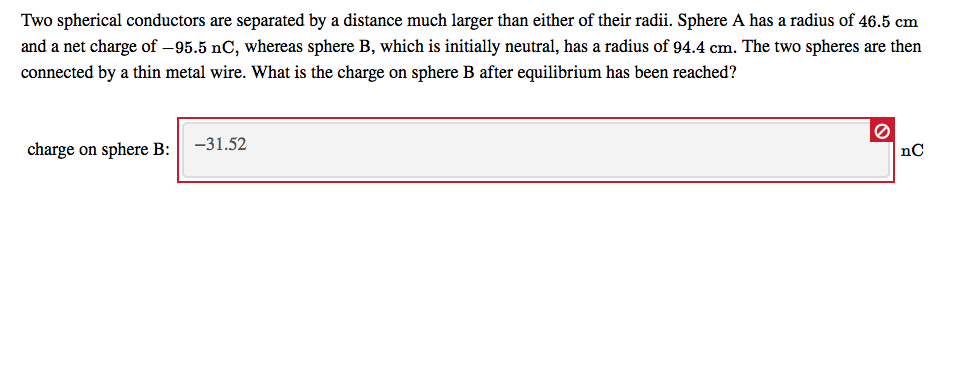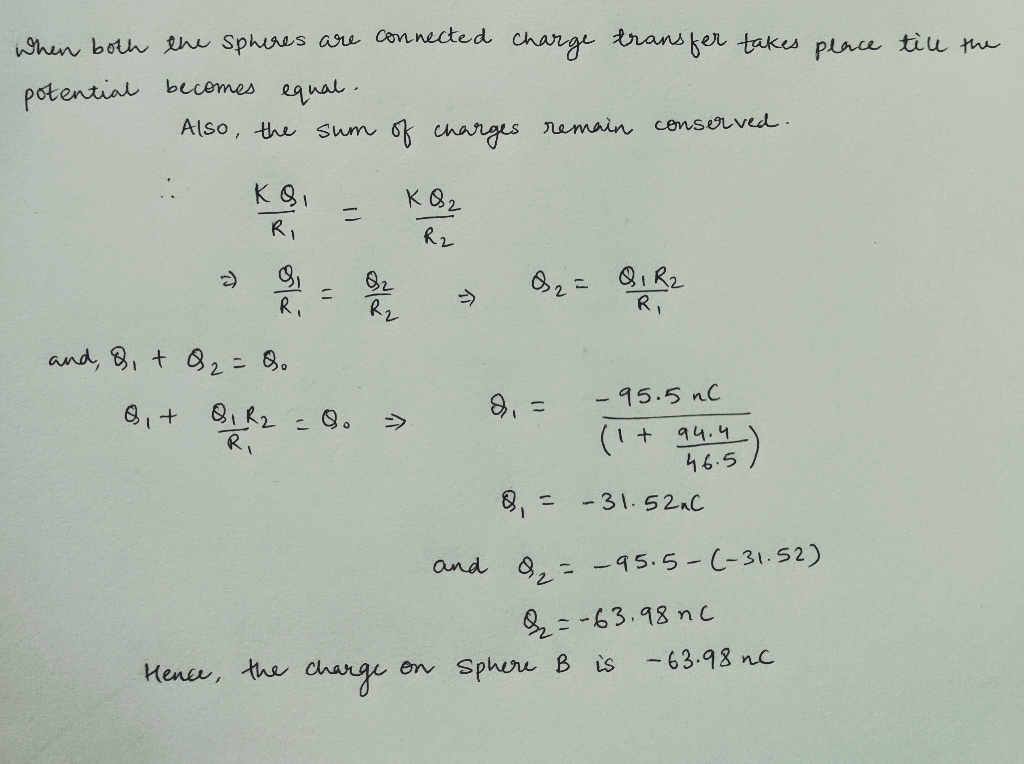#### Earn Coins

Coins can be redeemed for fabulous gifts.

Similar Homework Help Questions
• ### Two isolated conducting spheres are separated by a large distance. Sphere 1 has a radius of...

Two isolated conducting spheres are separated by a large distance. Sphere 1 has a radius of 20 cm and an initial charge 30 nC while Sphere 2 has a radius of 60 cm and an initial charge 70 nC. A very thin copper wire is now connected to the spheres to allow charge to ﬂow between them. How much charge will be transferred from Sphere 2 to Sphere 1? (Note that the charge transferred can be positive, negative or zero.)

• ### 4 Two spherical conductors (Homework #3) Two spherical conductors of different radii (Ri and R2) are...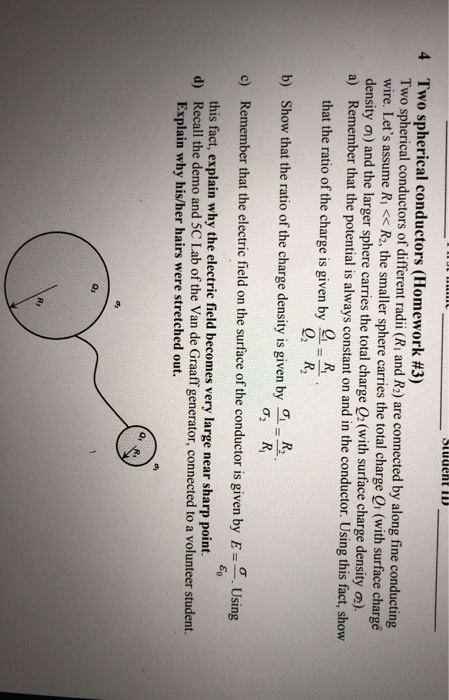4 Two spherical conductors (Homework #3) Two spherical conductors of different radii (Ri and R2) are connected by along fine conducting wire. Let's assume Ri << R2, the smaller sphere carries the total charge 0 (with surface chargé density o) and the larger sphere carries the total charge Q2 (with surface charge density ). a) Remember that the potential is always constant on and in the conductor. Using th is fact, show that the ratio of the charge is given...

• ### Two spherical conductors (Homework #3) Two spherical conductors of different radii (Ri and R:) are connected...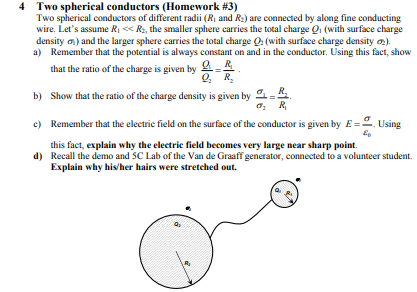Two spherical conductors (Homework #3) Two spherical conductors of different radii (Ri and R:) are connected by along fine conducting wire. Let's assume RiR, the smaller sphere carries the total charge Q (with surface charge density ) and the larger sphere carries the total charge a (with surface charge density a) Remember that the potential is always constant on and in the conductor. Using this fact, show that the ratio of the charge is given by R Q, R b)...

• ### You have two metal spheres, one with twice the diameter than the other. The smaller sphere...

You have two metal spheres, one with twice the diameter than the other. The smaller sphere initially has +8.0 nC of charge, while the larger one is uncharged. The two spheres are then connected together by a long, thin wire. What are the final charges on each sphere? Justify in words each relationship you use to solve this problem.

• ### Two spherical conductors A (radius 2.0 cm) and B (radius 3.0 cm) have initial charges of...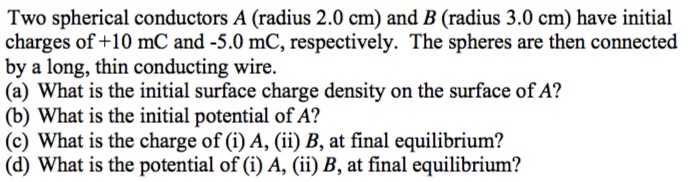Two spherical conductors A (radius 2.0 cm) and B (radius 3.0 cm) have initial charges of +10 mC and-5.0 mC, respectively. The spheres are then connected by a long, thin conducting wire (a) What is the initial surface charge density on the surface of A? (b) What is the initial potential of A? (c) What is the charge of (i) A, (ii) B, at final equilibrium? (d) What is the potential of (i) A, ii) B, at final equilibrium?

• ### (Concentric Spherical Conductors) due in an hour

A solid metal sphere of radius a = 2.2 cm has a net charge Qin =-3.4 nC (1 nC = 10-9C). Thesphere is surrounded by a concentricconducting spherical shell ofinner radius b = 6 cm and outer radius c = 9 cm.The shell has a net charge Qout = 2.3 nC. What isV0, theelectric potential at the center of the metal sphere, given thepotential at infinity is zero?V0 =V

• ### 4. Two conducting spheres are far apart. The smaller sphere has radius R and carries a...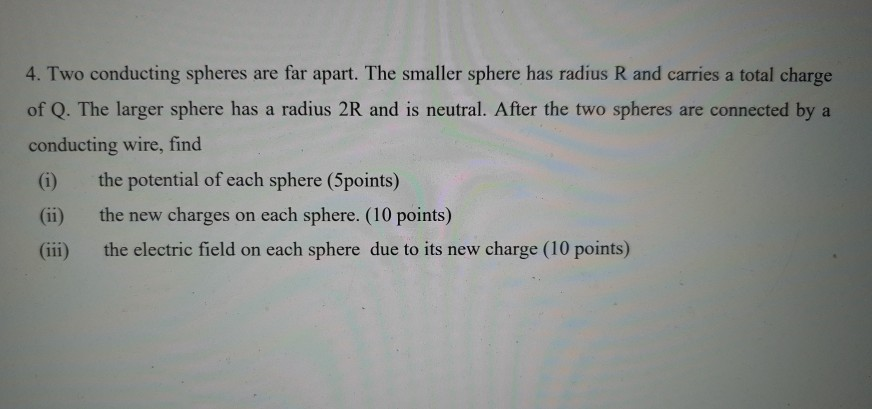4. Two conducting spheres are far apart. The smaller sphere has radius R and carries a total charge of Q. The larger sphere has a radius 2R and is neutral. After the two spheres are connected by a conducting wire, find the potential of each sphere (5points) the new charges on each sphere. (10 points) (iii) the electric field on each sphere due to its new charge (10 points)

• ### 4. Two conducting spheres are far apart. The smaller sphere has radius R and carries a...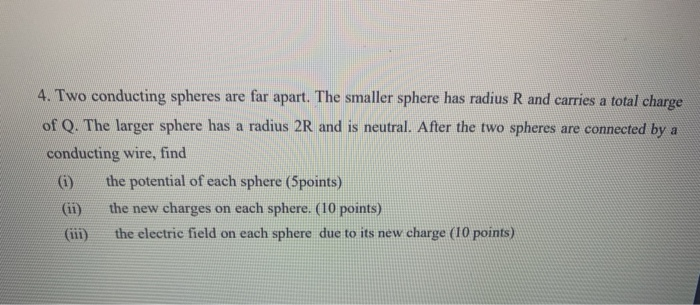4. Two conducting spheres are far apart. The smaller sphere has radius R and carries a total charge of Q. The larger sphere has a radius 2R and is neutral. After the two spheres are connected by a conducting wire, find (1) the potential of each sphere (5points) the new charges on each sphere. (10 points) the electric field on each sphere due to its new charge (10 points)

• ### 4. Two conducting spheres are far apart. The smaller sphere has radius R and carries a...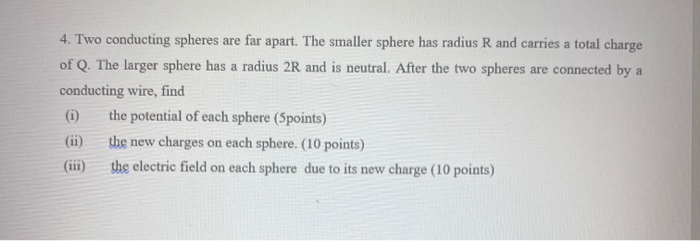4. Two conducting spheres are far apart. The smaller sphere has radius R and carries a total charge of Q. The larger sphere has a radius 2R and is neutral. After the two spheres are connected by a conducting wire, find (1) the potential of each sphere (Spoints) the new charges on each sphere. (10 points) (iii) the electric field on each sphere due to its new charge (10 points)

• ### 4. Two conducting spheres are far apart. The smaller sphere has radius R and carries a...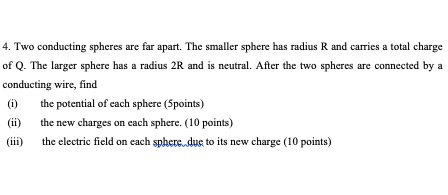4. Two conducting spheres are far apart. The smaller sphere has radius R and carries a total charge of Q. The larger sphere has a radius 2R and is neutral. After the two spheres are connected by a conducting wire, find (1) the potential of each sphere (Spoints) the new charges on each sphere. (10 points) (iii) the electric field on each sphere due to its new charge (10 points)